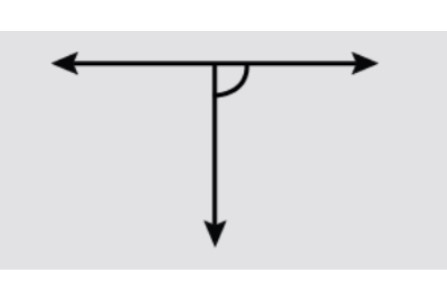Courses
Courses for Kids
Free study material
Offline Centres
MoreLast updated date: 24th Nov 2023
Total views: 381k
Views today: 9.81k

# Classify the following angles as right, straight, acute, obtuse, or reflex.Verified
381k+ views
Hint: Right angles are exclusively equal to $90{}^\circ$. Obtuse angles lie between $90{}^\circ$ and $180{}^\circ$. Acute angles lie between $0{}^\circ$ and $90{}^\circ$. Straight angles are exclusively equal to $180{}^\circ$. Reflex angles lie between $180{}^\circ$ and $360{}^\circ$.

Let’s start from backwards. Straight angle is an angle that is only equal to $180{}^\circ$. It can be thought of as the angle formed by a line, if we take any point on the line as the vertex of the angle. So, a straight angle is basically an angle where the vertex, and both the arms, lie on the same straight line. Over here, we can see that the vertex of the angle marked lies on the same line as both the arms, but the arms themselves are perpendicular to each other. Therefore, all the three don’t lie on the same straight line, and hence, the angle marked is not a straight angle.
Next, moving on to obtuse angles. Obtuse angles always lie between $90{}^\circ$ and $180{}^\circ$. The angle marked, can be seen is not more than $90{}^\circ$, hence, we can completely disregard the possibility of it being an obtuse angle.
Next, we have an acute angle. Acute angles lie between $0{}^\circ$ and $90{}^\circ$. We can also see from the figure, that the angle marked definitely is not smaller than $90{}^\circ$ either. So, it’s not an acute angle either.
Next, we have a reflex angle. A reflex angle lies between $180{}^\circ$ and $360{}^\circ$ always. We can also see that the angle marked does not look like the major sector of a circle. Hence, we can say that it’s not a reflex angle either.
However, till now, we have concluded that neither is it bigger than $90{}^\circ$, nor is it smaller than it. It, in fact, looks like it is exactly equal to $90{}^\circ$, and angles which are exclusively equal to $90{}^\circ$, are known as right angles.
Note: Don’t get confused between obtuse and reflex angle. To sum it up, if we consider any angle $x{}^\circ$, then it is acute when \$0